Question

A tank contains 90 kg of salt and 2000 L of water. Pure water enters a...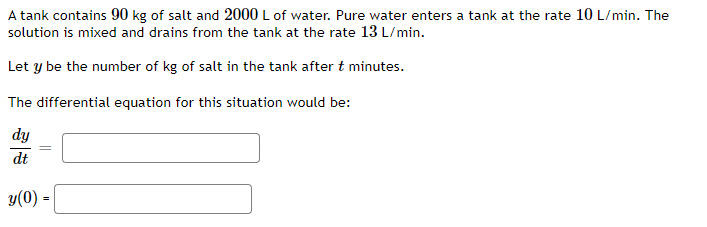A tank contains 90 kg of salt and 2000 L of water. Pure water enters a tank at the rate 10 L/min. The solution is mixed and drains from the tank at the rate 13 L/min. Let y be the number of kg of salt in the tank after t minutes. The differential equation for this situation would be: dy dt = y(0) -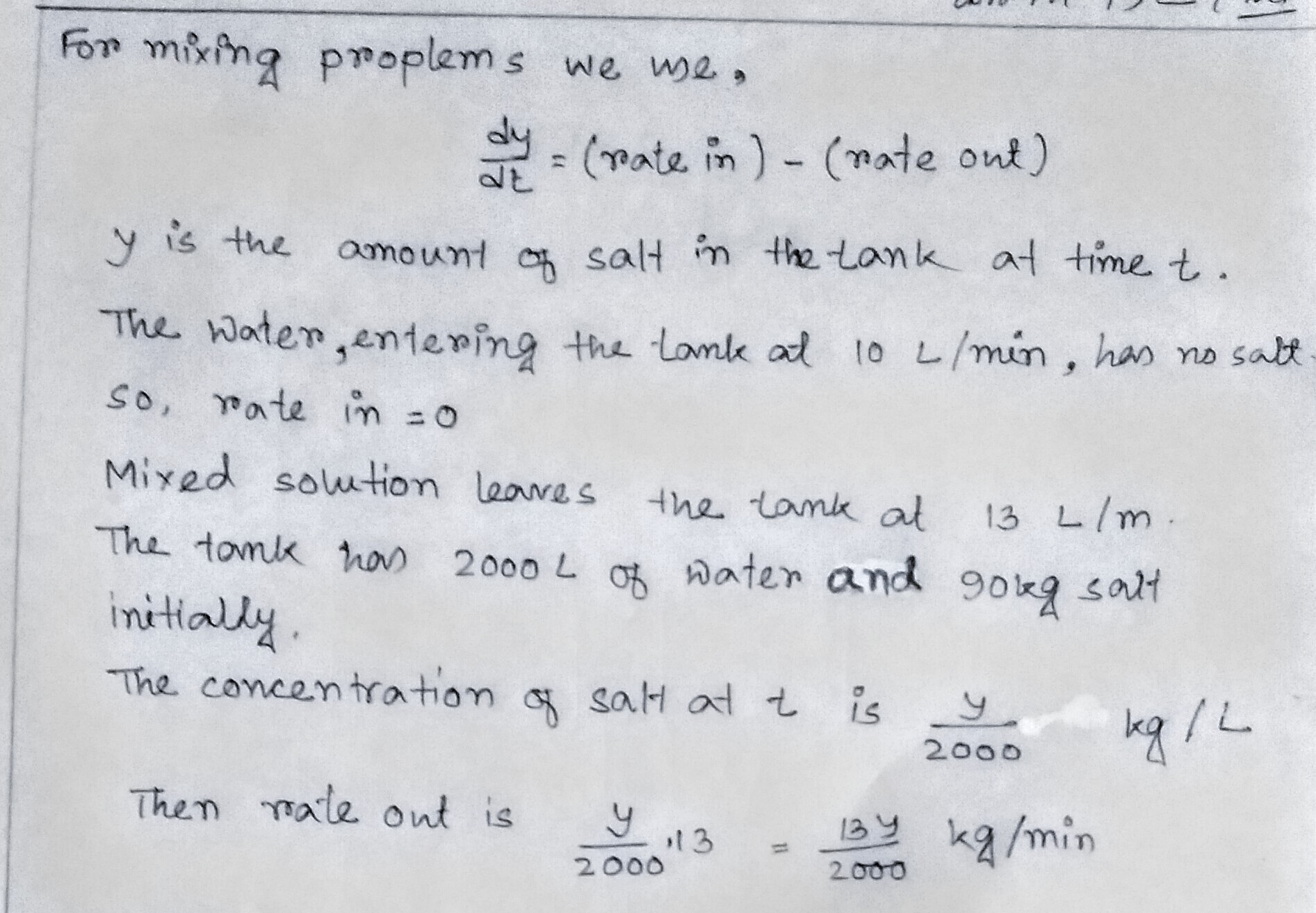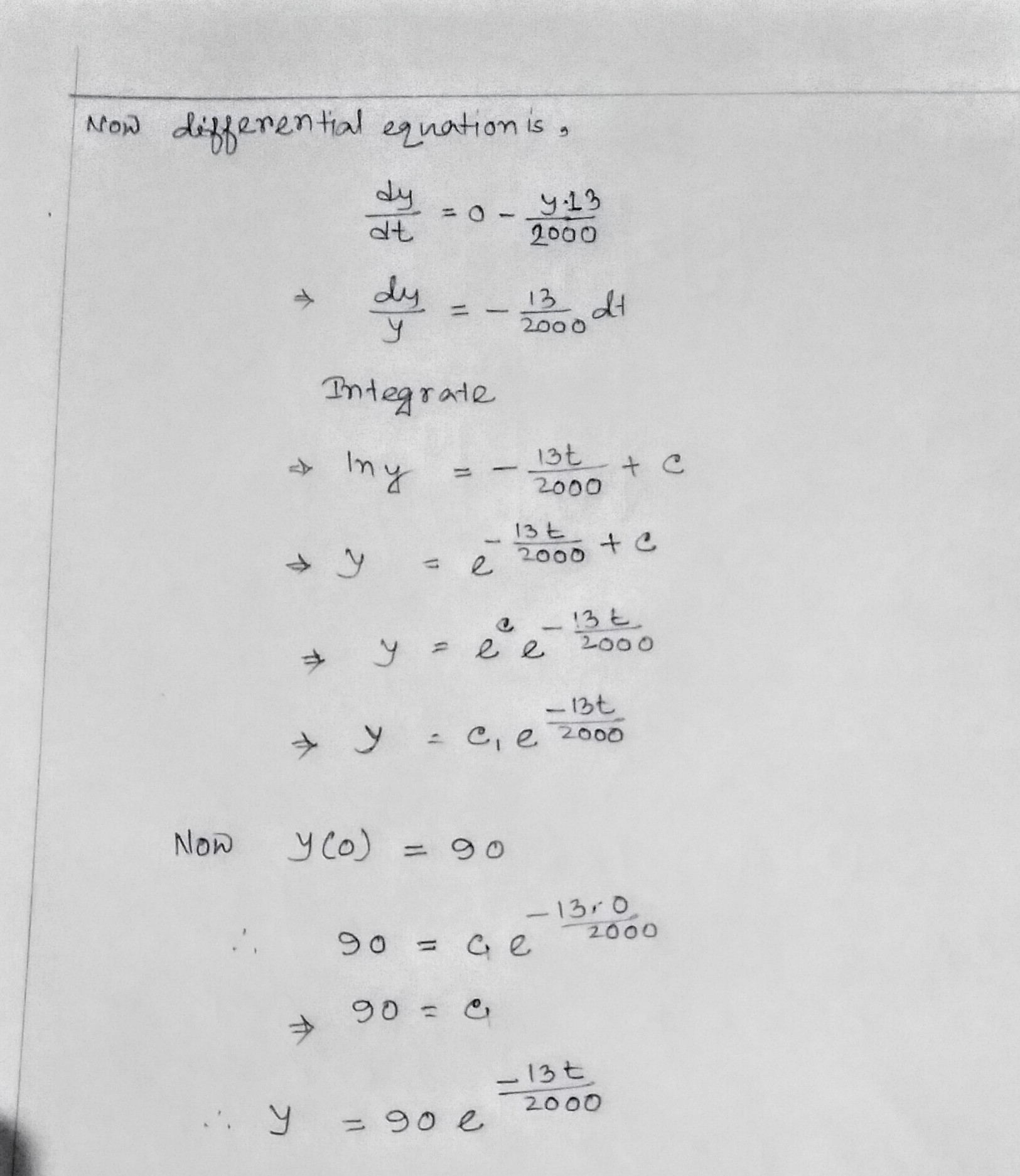Earn Coins

Coins can be redeemed for fabulous gifts.

Similar Homework Help Questions
• A tank contains 90 kg of salt and 2000 L of water. Pure water enters a...

A tank contains 90 kg of salt and 2000 L of water. Pure water enters a tank at the rate 6 L / min. The solution is mixed and drains from the tank at the rate 8 L / min.Let y be the number of kg of salt in the tank after t minutes.The differential equation for this situation would be:dy/dt=y(0)=

• a tank contains 60 kg of salt and 2000 L of water. pure water enters at...

a tank contains 60 kg of salt and 2000 L of water. pure water enters at 6L/min the solution is mixed and drains at 9L/min y=kg of salt after t minutes. dy/dt=??? and y(0)=???

• (1 pt) A tank contains 50 kg of salt and 2000 L of water. Pure water...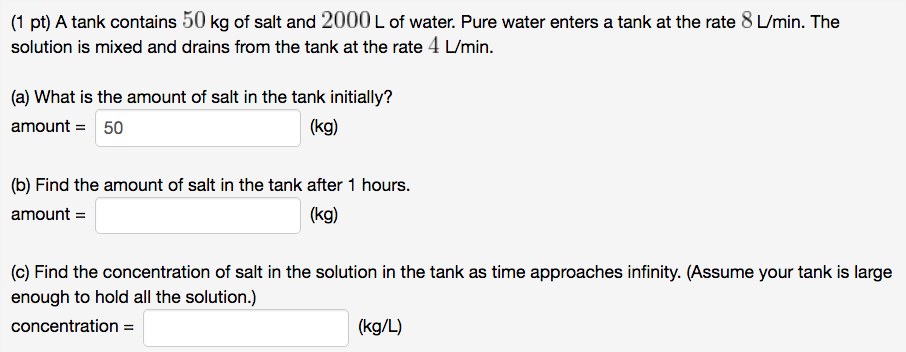(1 pt) A tank contains 50 kg of salt and 2000 L of water. Pure water enters a tank at the rate 8 L/min. The solution is mixed and drains from the tank at the rate 4 L/min. (a) What is the amount of salt in the tank initially? amount 50 (kg) (b) Find the amount of salt in the tank after 1 hours. (kg) amount (c) Find the concentration of salt in the solution in the tank as time...

• A tank contains 3,000 L of brine with 12 kg of dissolved salt. Pure water enters...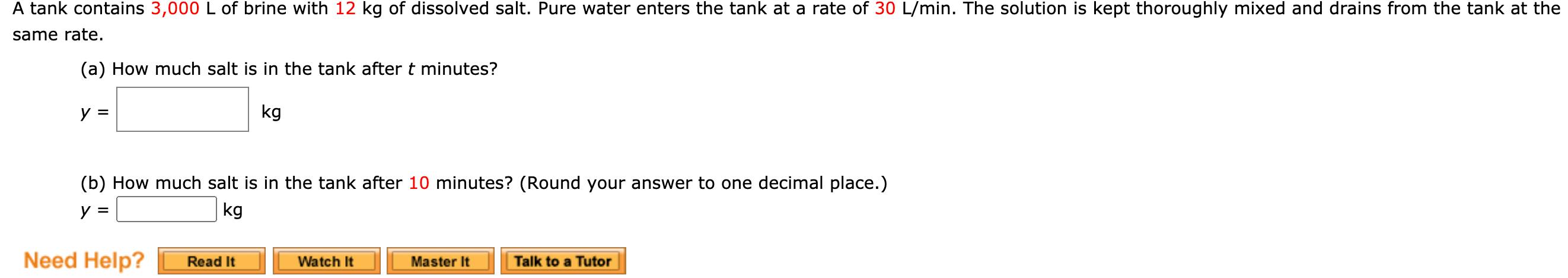A tank contains 3,000 L of brine with 12 kg of dissolved salt. Pure water enters the tank at a rate of 30 L/min. The solution is kept thoroughly mixed and drains from the tank at the same rate. (a) How much salt is in the tank after t minutes? y kg (b) How much salt is in the tank after 10 minutes? (Round your answer to one decimal place.) У kg Need Help? Read It Watch It Master It...

• A tank contains 70 kg of salt and 2000 L of water. Water containing 0.4kg/L of...

A tank contains 70 kg of salt and 2000 L of water. Water containing 0.4kg/L of salt enters the tank at the rate 16L/min. The solution is mixed and drains from the tank at the rate 4L/min. A(t) is the amount of salt in the tank at time t measured in kilograms. (a) A(0) =  (kg) (b) A differential equation for the amount of salt in the tank is  =0=0. (Use t,A, A', A'', for your variables, not A(t), and move everything...

• (1 point) A tank contains 50 kg of salt and 2000 L of water. A solution...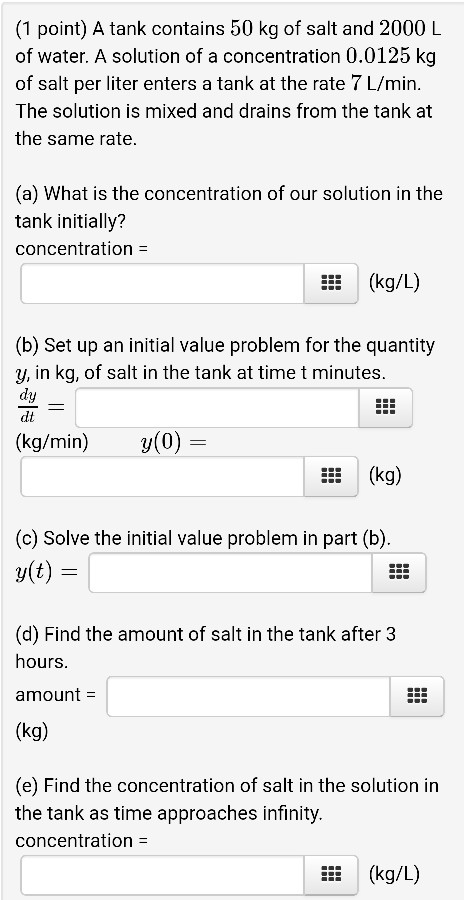(1 point) A tank contains 50 kg of salt and 2000 L of water. A solution of a concentration 0.0125 kg of salt per liter enters a tank at the rate 7 L/min. The solution is mixed and drains from the tank at the same rate. (a) What is the concentration of our solution in the tank initially? concentration = !!! (kg/L) (b) Set up an initial value problem for the quantity y, in kg, of salt in the tank...

• (1 point) A tank contains 70 kg of salt and 1000 L of water. Pure water...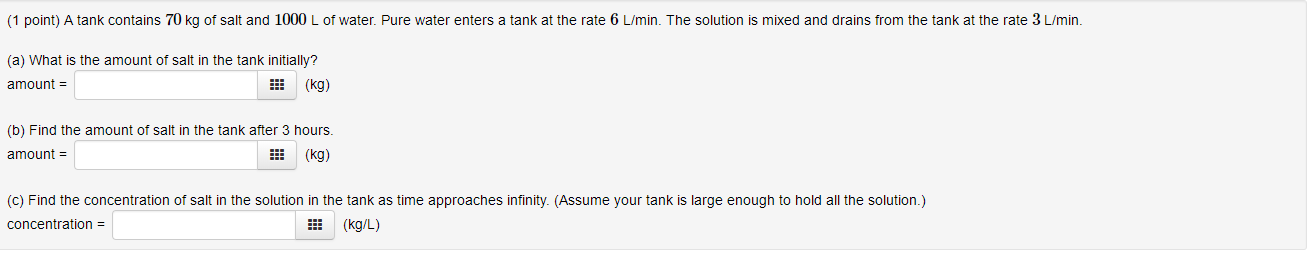(1 point) A tank contains 70 kg of salt and 1000 L of water. Pure water enters a tank at the rate 6 L/min. The solution is mixed and drains from the tank at the rate 3 L/min. (a) What is the amount of salt in the tank initially? amount = !!! (kg) (b) Find the amount of salt in the tank after 3 hours. amount = (kg) (c) Find the concentration of salt in the solution in the tank...

• (2 points) A tank contains 80 kg of salt and 1000 L of water. Pure water...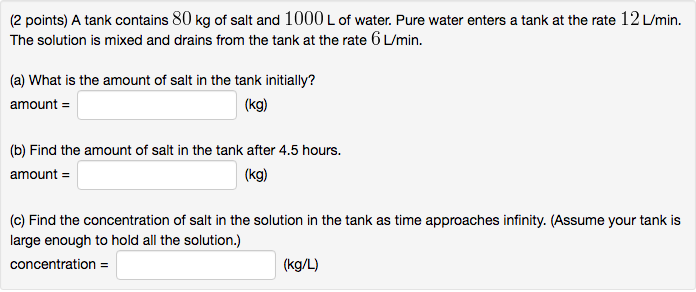(2 points) A tank contains 80 kg of salt and 1000 L of water. Pure water enters a tank at the rate 12 L/min. The solution is mixed and drains from the tank at the rate 6 L/min. (a) What is the amount of salt in the tank initially? amount = (kg) (b) Find the amount of salt in the tank after 4.5 hours. amount = (kg) (c) Find the concentration of salt in the solution in the tank as...

• Only need answer for b.). Please show your work! A tank contains 70 kg of salt and 1000 L of water. Pure water enters a...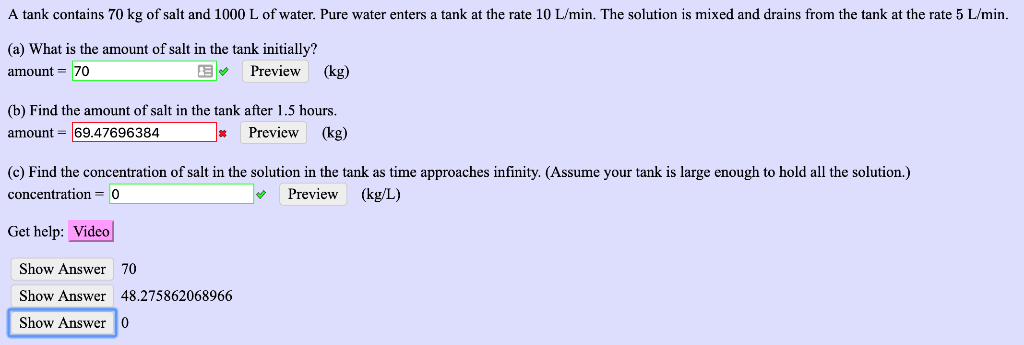Only need answer for b.). Please show your work! A tank contains 70 kg of salt and 1000 L of water. Pure water enters a tank at the rate 10 L/min. The solution is mixed and drains from the tank at the rate 5 L/min (a) What is the amount of salt in the tank initially? Preview (kg) amount-70 Find the amount of salt in the tank after 1.5 hours. * Preview (kg) amount - 69.47696384 (c) Find the concentration...

• A tank contains 15,000 L of brine with 23 kg of dissolved salt.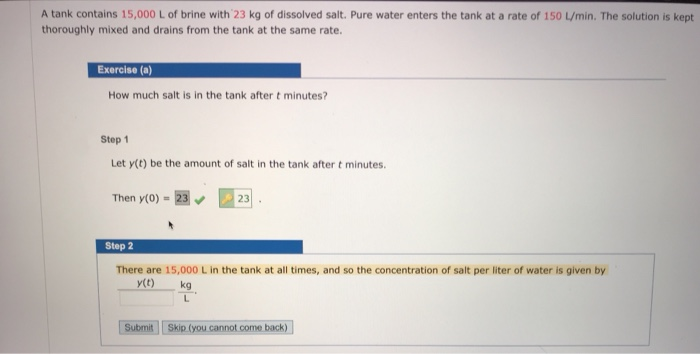A tank contains 15,000 L of brine with 23 kg of dissolved salt. Pure water enters the tank at a rate of 150 L / min. The solution is kept thoroughly mixed and drains from the tank at the same rate.Exereise (a)How much salt is in the tank after t minutes?Exercise (b)How much salt is in the tank after 10 minutes?